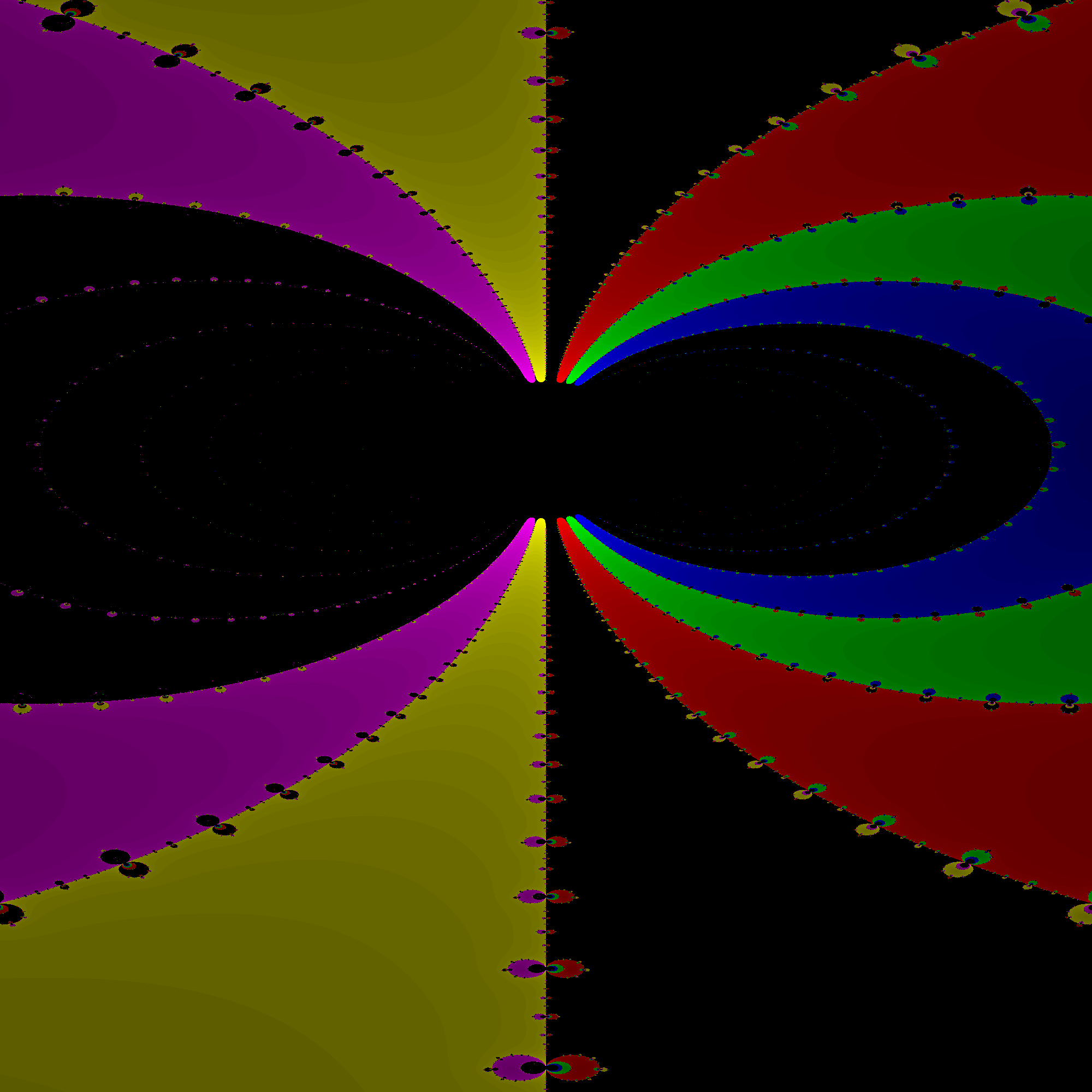This software uses Newton’s method (the one from any first year calculus course) in the complex plane to generate fractals. This code makes use of many of the new C++11 language features, so a newer compiler will be required (I used GCC 4.8.1 running on Opensuse). This code is documented thoroughly with doxygen.

## How it Works

A rectangular region is first chosen in the complex plane. Then, the fractal is computed row by row (a row is a line with all points on the line having a constant imaginary component – a horizontal line). Each point on the row is used as the initial guess for Newton’s method. Newton’s method will eventually converge on one of the zeros of the fractal generating function. The color of the associated pixel is chosen by which zero Newton’s method finds. The shading is derived from the number of iterations needed to reach the zero.

Here